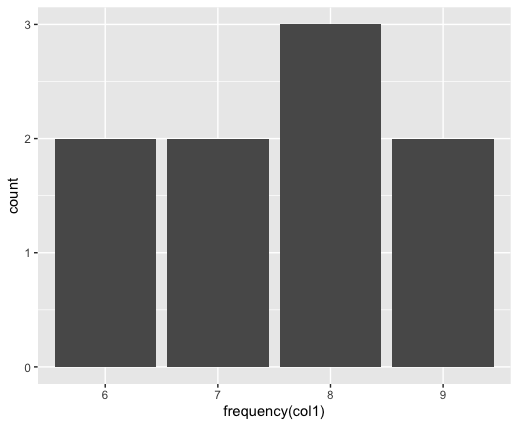# How to make a frequency distribution table in R ?

• Last Updated : 07 Sep, 2021

In this article, we are going to see how to make a frequency distribution table using R Programming Language.

The table() method in R is used to compute the frequency counts of the variables appearing in the specified column of the dataframe. The result is returned to the form of a two-row tabular structure, where the first row indicates the value of the column and the next indicates its corresponding frequencies.

`frq-table <- table (x), where x is the data.table object`

The cumulative frequency distribution of a given data set is the summation of all the classes including this class below it in a frequency distribution table obtained. The value at any cell position is obtained by the summation of all the previous values and the current value encountered till now. The cumsum() function can be used to calculate this.

`cumsum( frq-table) `

Relative frequency also known as the probability distribution, is the frequency of the corresponding value divided by the total number of elements. This can be calculated by either prop.table() method applied over the frequency table.

```prop.table (frq-table)
OR
frq-table / total observations```

Example 1: Making a frequency distribution table.

## R

 `library ``(``'data.table'``)` `# creating a dataframe``data_table <- ``data.table``(col1 = ``sample``(6 : 9, 9 ,``                                       ``replace = ``TRUE``),``                         ` `                         ``col2 = ``letters``[1 : 3],``                         ` `                         ``col3 = ``c``(1, 4, 1, 2, 2,``                                  ``2, 1, 2, 2))``print ``(``"Original DataFrame"``)``print ``(data_table)` `freq <- ``table``(data_table\$col1)` `print ``(``"Modified Frequency Table"``)``print ``(freq)` `print ``(``"Cumulative Frequency Table"``)``cumsum <- ``cumsum``(freq)``print ``(cumsum)` `print ``(``"Relative Frequency Table"``)``prob <- ``prop.table``(freq)``print ``(prob)`

Output

``` "Original DataFrame"
col1 col2 col3
1:    9    a    1
2:    6    b    4
3:    6    c    1
4:    6    a    2
5:    8    b    2
6:    7    c    2
7:    6    a    1
8:    6    b    2
9:    8    c    2
 "Modified Frequency Table"
6 7 8 9
5 1 2 1
 "Cumulative Frequency Table"
6 7 8 9
5 6 8 9
 "Relative Frequency Table"
6      7      8      9
0.5556 0.1111 0.2222 0.1111 ```

Example 2:

The table() method can accept multiple arguments as its parameters. All the combinations are generated by the cross joining of the column values and then the unique elements are extracted, and their corresponding frequency counts too. The result is obtained in the form of 2D matrix with the number of rows and column equivalent to all combinations and matrix cell values as its counts.

## R

 `library ``(``'data.table'``)` `# creating a dataframe``data_table <- ``data.table``(col1 = ``sample``(6 : 9, 9 ,``                                       ``replace = ``TRUE``),``                         ``col2 = ``letters``[1 : 3],``                         ` `                         ``col3 = ``c``(1, 4, 1, 2, 2,``                                  ``2, 1, 2, 2))``print ``(``"Original DataFrame"``)``print ``(data_table)` `freq <- ``table``(data_table\$col1, data_table\$col3)``print ``(``"Modified Frequency Table"``)``print ``(freq)`

Output

``` "Original DataFrame"
col1 col2 col3
1:    7    a    1
2:    7    b    4
3:    7    c    1
4:    8    a    2
5:    9    b    2
6:    9    c    2
7:    9    a    1
8:    7    b    2
9:    9    c    2
 "Modified Frequency Table"
1 2 4
6 2 1 0
7 1 0 0
8 0 1 1
9 0 3 0```

Example 3:

The data frequency and cumulative frequency tables can also be visualized by importing the data set into the working space. The frequency table is plotted to keep col1 of the dataframe in mind. The frequency counts of each of the values are plotted indicating the number of its total occurrences.

## R

 `# import libraries``library``(``"ggplot2"``)` `# creating a dataframe``data_table <- ``data.frame``(col1 = ``sample``(6 : 9, 9 ,``                                       ``replace = ``TRUE``),``                         ``col2 = ``letters``[1:3],``                         ``col3 = ``c``(1, 4, 1, 2,``                                  ``2, 2, 1, 2, 2))``print ``(``"Original DataFrame"``)``print ``(data_table)` `# computing frequency``freq_tbl <- ``table``(data_table\$col1)``print ``(``"Frequency count"``)``print ``(freq_tbl)` `# re-order levels``frequency <- ``function``(x) {``  ``factor``(x, levels = ``names``(``table``(x)))``}` `# plotting the data with the help of ggplot``ggplot``(data_table, ``aes``(x = ``frequency``(`col1`))) +``  ``geom_bar``()`

Output

``` "Original DataFrame"
col1 col2 col3
1    6    a    1
2    9    b    4
3    6    c    1
4    9    a    2
5    8    b    2
6    8    c    2
7    8    a    1
8    7    b    2
9    7    c    2```My Personal Notes arrow_drop_up